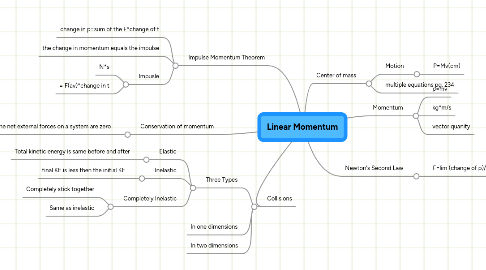# Linear Momentum

Get Started. It's FreeLinear Momentum## 1. Impulse Momentum Theorem

### 1.3. Impusle

1.3.1. N*s

1.3.2. = F(av)*change in t

## 3. Collisions

### 3.1. Three Types

3.1.1. Elastic

3.1.1.1. Total kinetic energy is same before and after

3.1.2. Inelastic

3.1.2.1. final KE is less then the initial KE

3.1.3. Completely Inelastic

3.1.3.1. Completely stick together

3.1.3.2. Same as inelastic

6.1.1. P=Mv(cm)